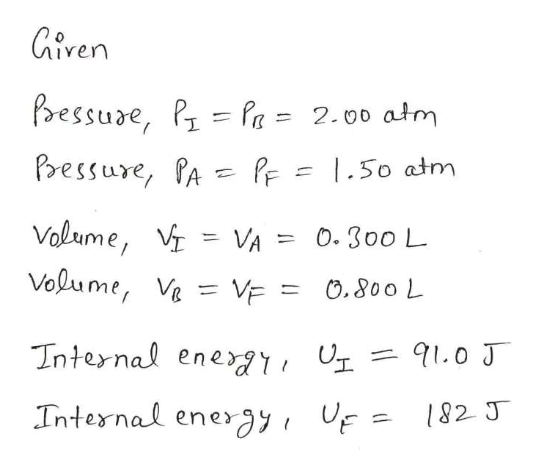# Two moles of gas initially at a pressure of 2.00 atm and a volume of 0.300 L has internal energy equal to 91.0 J. In its final state, the gas is at a pressure of 1.50 atm and a volume of 0.800 L, and its internal energy equals 182 J.For the paths IAF, IBF, and IF in the figure above, calculate the work done on the gas.a. W(IAF)b. W(IF)c. Q(IAF)d. Q(IBF)e. (QIF)

Question
7 views

Two moles of gas initially at a pressure of 2.00 atm and a volume of 0.300 L has internal energy equal to 91.0 J. In its final state, the gas is at a pressure of 1.50 atm and a volume of 0.800 L, and its internal energy equals 182 J.

For the paths IAFIBF, and IF in the figure above, calculate the work done on the gas.

a. W(IAF)

b. W(IF)

c. Q(IAF)

d. Q(IBF)

e. (QIF)

check_circle

Step 1

Since we are entitled to answer up to 3 sub-parts, we’ll answer the first 3 as you have not
mentioned the subparts you need help with. Please resubmit the question and specify the other
subparts you’d like to get answered.

Step 2
Step 3help_outlineImage TranscriptioncloseGiren bessure, PPa = 2.00 atm Pressure, PA PF = |.50 atm Volume, VA Volume, Ve = VE = = 0. 300 L O.800 L 91.0 J Internal ene Internal energy F = 182 J fullscreen

### Want to see the full answer?

See Solution

#### Want to see this answer and more?

Solutions are written by subject experts who are available 24/7. Questions are typically answered within 1 hour.*

See Solution
*Response times may vary by subject and question.
Tagged in

### Thermodynamics